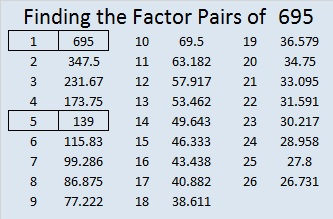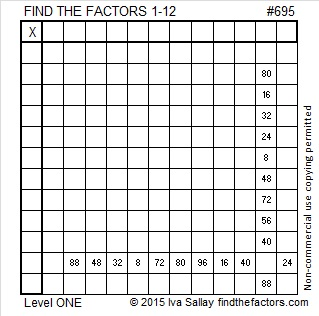# 695 and Level 1

• 695 is a composite number.
• Prime factorization: 695 = 5 x 139
• The exponents in the prime factorization are 1 and 1. Adding one to each and multiplying we get (1 + 1)(1 + 1) = 2 x 2 = 4. Therefore 695 has exactly 4 factors.
• Factors of 695: 1, 5, 139, 695
• Factor pairs: 695 = 1 x 695 or 5 x 139
• 695 has no square factors that allow its square root to be simplified. √695 ≈ 26.36285.Here is today’s puzzle:Print the puzzles or type the solution on this excel file: 12 Factors 2015-11-30

———————————————————————————

Here are a few more facts about the number 695:

695 is the sum of the eighteen prime numbers from 7 to 73.

695 is also the hypotenuse of the Pythagorean triple 417-556-695. What is the greatest common factor of those three numbers?

137 + 138 + 139 + 140 + 141 = 695 so it is the sum of 5 consecutive numbers.

Since 695 is odd, it is the sum of two consecutive numbers, 347 and 348.

———————————————————————————This site uses Akismet to reduce spam. Learn how your comment data is processed.